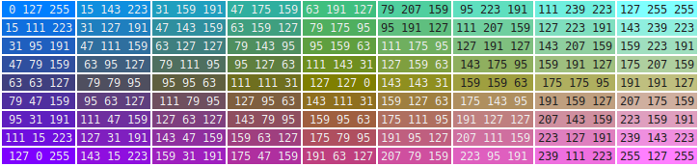## A Colorful Table

In another simple example, we dynamically generate a table selecting a different background color for each cell.:

```puts "<html><head>"
puts "<style>\n  td { font-size: 12px; }"
puts "td.bright { color: #eee; }"
puts "td.dark { color: #222; }\n </style>"
puts "<table>"

# we create a 8x8 table selecting a different background for each cell

for {set i 0} { \$i < 9 } {incr i} {
puts "<tr>"
for {set j 0} {\$j < 9} {incr j} {

set r [expr int(255 * (\$i + \$j) / 16)]
set g [expr int(255 * (8 - \$i + \$j) / 16)]
set b [expr int(255 * (\$i + 8 - \$j) / 16)]

# determining the background luminosity (YIQ space of NTSC) and choosing
# the foreground color accordingly in order maintain maximum contrast

if { [expr (\$r*0.29894)+(\$g*0.58704)+(\$b*0.11402)] > 128} {
set cssclass "dark"
} else {
set cssclass "bright"
}

puts [format "<td bgcolor=\"%02x%02x%02x\" class=\"%s\">\$r \$g \$b</td>" \$r \$g \$b \$cssclass]
}
puts "</tr>"
}
puts "</table>"
puts "</body></html>"```

The text color is determined through a simple CSS rule we put within a HTML <style> element. Create a file (e.g. color-table.tcl) and put the following code in it. If you read the code, you can see that this is pure Tcl. We could take the same code, run it outside of Rivet, and it would generate the same HTML!

The result should look something like this: